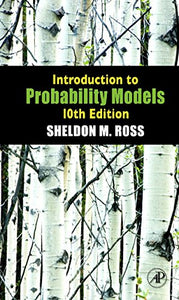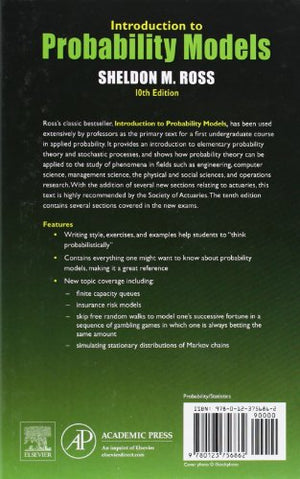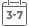# Introduction to Probability Models, Tenth Edition

• Publish Date: 2009-12-17
• Binding: Hardcover
• Author: Sheldon M. Ross
Regular price \$110.46 Sale price \$43.46Introduction to Probability Models, Tenth Edition, provides an introduction to elementary probability theory and stochastic processes. There are two approaches to the study of probability theory.

One is heuristic and nonrigorous, and attempts to develop in students an intuitive feel for the subject that enables him or her to think probabilistically. The other approach attempts a rigorous development of probability by using the tools of measure theory. The first approach is employed in this text.

The book begins by introducing basic concepts of probability theory, such as the random variable, conditional probability, and conditional expectation. This is followed by discussions of stochastic processes, including Markov chains and Poison processes. The remaining chapters cover queuing, reliability theory, Brownian motion, and simulation. Many examples are worked out throughout the text, along with exercises to be solved by students.

This book will be particularly useful to those interested in learning how probability theory can be applied to the study of phenomena in fields such as engineering, computer science, management science, the physical and social sciences, and operations research. Ideally, this text would be used in a one-year course in probability models, or a one-semester course in introductory probability theory or a course in elementary stochastic processes.

New to this Edition:

• 65% new chapter material including coverage of finite capacity queues, insurance risk models and Markov chains
• Contains compulsory material for new Exam 3 of the Society of Actuaries containing several sections in the new exams
• Updated data, and a list of commonly used notations and equations, a robust ancillary package, including a ISM, SSM, and test bank
• Includes SPSS PASW Modeler and SAS JMP software packages which are widely used in the field

Hallmark features:

• Superior writing style
• Excellent exercises and examples covering the wide breadth of coverage of probability topics
• Real-world applications in engineering, science, business and economics